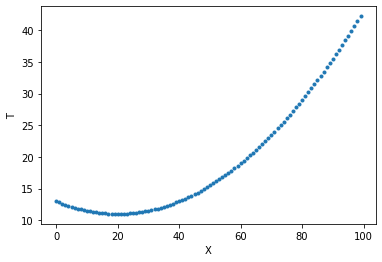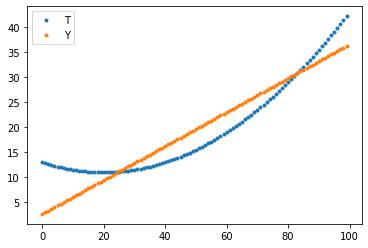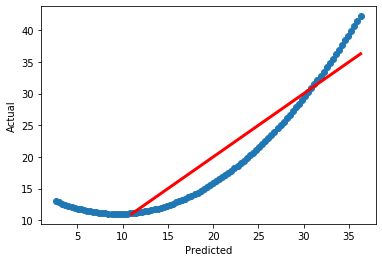# A1.1 Linear Regression with SGD¶

In this assignment, you will implement three functions train, use, and rmse and apply them to some weather data. Here are the specifications for these functions, which you must satisfy.

model = train(X, T, learning_rate, n_epochs, verbose)

• X: is an $N$ x $D$ matrix of input data samples, one per row. $N$ is the number of samples and $D$ is the number of variable values in each sample.
• T: is an $N$ x $K$ matrix of desired target values for each sample. $K$ is the number of output values you want to predict for each sample.
• learning_rate: is a scalar that controls the step size of each update to the weight values.
• n_epochs: is the number of epochs, or passes, through all $N$ samples, to take while updating the weight values.
• verbose: is True or False (default value) to control whether or not occasional text is printed to show the training progress.
• model: is the returned value, which must be a dictionary with the keys 'w', 'Xmeans', 'Xstds', 'Tmeans' and 'Tstds'.

Y = use(X, model)

• X: is an $N$ x $D$ matrix of input data samples, one per row, for which you want to predict the target values.
• model: is the dictionary returned by train.
• Y: is the returned $N$ x $K$ matrix of predicted values, one for each sample in X.

result = rmse(Y, T)

• Y: is an $N$ x $K$ matrix of predictions produced by use.
• T: is the $N$ x $K$ matrix of target values.
• result: is a scalar calculated as the square root of the mean of the squared differences between each sample (row) in Y and T.

To get you started, here are the standard imports we need.

In [ ]:
import numpy as np
import matplotlib.pyplot as plt
import pandas


## 60 points: 40 for train, 10 for use, 10 for rmse¶

Now here is a start at defining the train, use, and rmse functions. Fill in the correct code wherever you see . . . with one or more lines of code.

In [ ]:
def train(X, T, learning_rate, n_epochs, verbose=False):

# Calculate means and standard deviations of each column in X and T
. . .

# Use the means and standard deviations to standardize X and T
. . .

# Insert the column of constant 1's as a new initial column in X
. . .

# Initialize weights to be a numpy array of the correct shape and all zeros values.
. . .

for epoch in range(n_epochs):
sqerror_sum = 0

for n in range(n_samples):

# Use current weight values to predict output for sample n, then
# calculate the error, and
# update the weight values.
. . .

# Add the squared error to sqerror_sum
. . .

if verbose and (n_epochs < 11 or (epoch + 1) % (n_epochs // 10) == 0):
rmse = np.sqrt(sqerror_sum / n_samples)
rmse = rmse[0, 0]  # because rmse is 1x1 matrix
print(f'Epoch {epoch + 1} RMSE {rmse:.2f}')

return {'w': w, 'Xmeans': Xmeans, 'Xstds': Xstds,
'Tmeans': Tmeans, 'Tstds': Tstds}

In [ ]:
def use(X, model):
# Standardize X using Xmeans and Xstds in model
. . .
# Predict output values using weights in model
. . .
# Unstandardize the predicted output values using Tmeans and Tstds in model
. . .
# Return the unstandardized output values

In [ ]:
def rmse(A, B):
. . .


In :
X = np.arange(0, 100).reshape(-1, 1)  # make X a 100 x 1 matrix
T = 0.5 + 0.3 * X + 0.005 * (X - 50) ** 2
plt.plot(X, T, '.')
plt.xlabel('X')
plt.ylabel('T');In :
model = train(X, T, 0.01, 50, verbose=True)
model

Epoch 5 RMSE 0.40
Epoch 10 RMSE 0.40
Epoch 15 RMSE 0.40
Epoch 20 RMSE 0.40
Epoch 25 RMSE 0.40
Epoch 30 RMSE 0.40
Epoch 35 RMSE 0.40
Epoch 40 RMSE 0.40
Epoch 45 RMSE 0.40
Epoch 50 RMSE 0.40

Out:
{'w': array([[-0.00576098],
[ 1.05433338]]),
'Xmeans': array([49.5]),
'Xstds': array([28.86607005]),
'Tmeans': array([19.5175]),
'Tstds': array([9.29491938])}
In :
Y = use(X, model)
plt.plot(T, '.', label='T')
plt.plot(Y, '.', label='Y')
plt.legend()

Out:
<matplotlib.legend.Legend at 0x7fdcaad26fd0>In :
plt.plot(Y[:, 0], T[:, 0], 'o')
plt.xlabel('Predicted')
plt.ylabel('Actual')
a = max(min(Y[:, 0]), min(T[:, 0]))
b = min(max(Y[:, 0]), max(T[:, 0]))
plt.plot([a, b], [a, b], 'r', linewidth=3)

Out:
[<matplotlib.lines.Line2D at 0x7fdcaad61dd0>]## Weather Data¶

Now that your functions are working, we can apply them to some real data. We will use data from CSU's CoAgMet Station Daily Data Access.

You can get the data file here

## 5 points:¶

Read in the data into variable df using pandas.read_csv like we did in lecture notes. Missing values in this dataset are indicated by the string '***'.

In [ ]:
...


## 5 points:¶

Check for missing values by showing the number of NA values, as shown in lecture notes.

In [ ]:
...


## 5 points:¶

If there are missing values, remove samples that contain missing values. Prove that you were successful by counting the number of missing values now, which should be zero.

In [ ]:
...


Your job is now to create a linear model that predicts the next day's average temperature (tave) from the previous day's values of

1. tave: average temperature
2. tmax: maximum temperature
3. tmin: minimum temperature
4. vp: vapor pressure
5. rhmax: maximum relative humidity
6. rhmin: minimum relative humidity
7. pp: precipitation
8. gust: wind gust speed

As a hint on how to do this, here is a list with these column names:

In [ ]:
Xnames = ['tave', 'tmax', 'tmin', 'vp', 'rhmax', 'rhmin', 'pp', 'gust']
Tnames = ['next tave']


## 5 points:¶

Now select those eight columns from df and convert the result to a numpy array. (Easier than it sounds.) Then assign X to be all columns and all but the last row. Assign T to be just the first column (tave) and all but the first sample. So now the first row (sample) in X is associated with the first row (sample) in T which tave for the following day.

In [ ]:
...


## 15 points:¶

Use the function train to train a model for the X and T data. Run it several times with different learning_rate and n_epochs values to produce decreasing errors. Use the use function and plots of T versus predicted Y values to show how well the model is working. Type your observations of the plot and of the value of rmse to discuss how well the model succeeds.

In [ ]:
...


## 5 points:¶

Print the weight values in the resulting model along with their corresponding variable names (in Xnames). Use the relative magnitude of the weight values to discuss which input variables are most significant in predicting the changes in the tave values.

In [ ]:
...


Your notebook will be partially run and graded automatically. Test this grading process by first downloading A1grader.zip and extract A1grader.py from it. Run the code in the following cell to demonstrate an example grading session. You should see a perfect execution score of 60/60 if your functions are defined correctly. The remaining 40 points will be based on other testing and the results you obtain and your discussions.

A different, but similar, grading script will be used to grade your checked-in notebook. It will include additional tests. You should design and perform additional tests on all of your functions to be sure they run correctly before checking in your notebook.

For the grading script to run correctly, you must first name this notebook as Lastname-A1.ipynb with Lastname being your last name, and then save this notebook and check it in at the A1 assignment link in our Canvas web page.

In :
%run -i A1grader.py

======================= Code Execution =======================

Extracting python code from notebook named 'Instructor-A1.ipynb' and storing in notebookcode.py
Removing all statements that are not function or class defs or import statements.

Testing
X = np.array([1, 2, 3, 4, 5, 8, 9, 11]).reshape((-1, 1))
T = (X - 5) * 0.05 + 0.002 * (X - 8)**2
model = train(X, T, 0.001, 1000, True)

Epoch 100 RMSE 0.46
Epoch 200 RMSE 0.24
Epoch 300 RMSE 0.15
Epoch 400 RMSE 0.13
Epoch 500 RMSE 0.13
Epoch 600 RMSE 0.12
Epoch 700 RMSE 0.12
Epoch 800 RMSE 0.12
Epoch 900 RMSE 0.12
Epoch 1000 RMSE 0.12

--- 20/20 points. Returned correct values.

--- 10/10 points. Xmeans and Xstds are correct values.

--- 10/10 points. Tmeans and Tstds are correct values.

Testing
Y = use(X, model)

--- 10/10 points. Returned correct values.

Testing
err = rmse(Y, T)

--- 10/10 points. Returned correct values.

======================================================================
instructor Execution Grade is 60 / 60
======================================================================

__ / 5 Reading in weather.data correctly.

__ / 5 Count missing values, to show there are some.

__ / 5 Remove samples with missing values. Count to show there are none.

__ / 5 Construct X and T matrices as specified.

__ / 15 Use your train function on X and T. Show results as RMSE values and as plots
for several different values of learning_rate and n_epochs. Type your
observations of RMSE values and of plots with at least five sentences.

__ / 5 Print the weight values with corresponding variable names. Discuss
which input variablesa re most signficant in predicting tave values.

======================================================================
instructor FINAL GRADE is  _  / 100
======================================================================

Extra Credit:
Predict the change in tave from one day to the next, instead of the actual tave.
Show and discuss RMSE and plotting results for several values of learning_rate
and n_epochs.

instructor EXTRA CREDIT is 0 / 1


## Extra Credit: 1 point¶

A typical problem when predicting the next value in a time series is that the best solution may be to predict the previous value. The predicted value will look a lot like the input tave value shifted on time step later.

To do better, try predicting the change in tave from one day to the next. T can be assigned as

In [ ]:
T = data[1:, 0:1] -  data[:-1, 0:1]


Now repeat the training experiments to pick good learning_rate and n_epochs. Use predicted values to produce next day tave values by adding the predicted values to the previous day's tave. Use rmse to determine if this way of predicting next tave is better than directly predicting tave.